Study Guide

## Electric Potential

If electric fields can create forces and move charges around, they must be able to change charged particles' mechanical energies. This is true—and to that end, in this section we'll meet another form of potential energy, called electric potential energy (Ue). To get there, though, we first must be introduced to a new concept: electric potential (V).

This is a lot like meeting identical twins who immediately get offended when you confuse them; however, even though the difference between "electric potential energy" and "electric potential" is etymologically small, they're totally different people and it's important not to confuse the two.

Electric potential—often called electric potential difference or voltage—is the potential energy that a theoretical +1 C charge would have if placed in an existing electric field. Electric potential energy is the potential energy the charge you're concerned with actually possesses. In other words, for a charge q in a field E with associated potential V:

Ue = qV

You can think of the electric potential of a charge as a measure of how hard it is to push another charged particle near it (because of Coulomb's Law, we know like charges are going to resist being close to each other). This is the amount of work you'd have to do to move a charged particle per unit of charge, and so it's measured in joules per coulomb, which is defined in SI units as a volt (V). The potential created by a charge q is given by: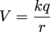We can combine these two formulas to get the potential energy a charge q1 possesses when it's moved to a distance r from charge q2: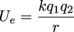So you can see that there's a circle of radius r around charge q2 where the energy needed to move q1 to any point on the circle is exactly the same—every point on the circle is a distance r away from q2. We can draw this on our field line diagram, where it's called an equipotential line.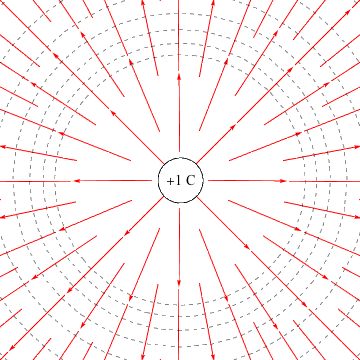In fact, equipotential lines are easy to add to any field line diagram. They intersect field lines at a 90º angle—always—and are closer together where the field is stronger.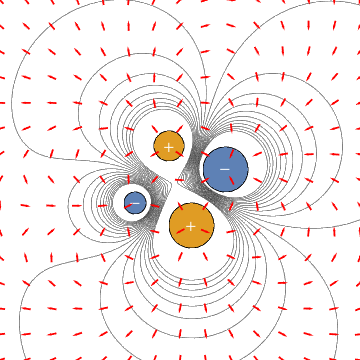These field line and equipotential line drawings may look a lot like topographic maps—this is a perfectly fine analogy. Just like gravity makes it hard to roll a heavy ball up a steep hill, the Coulomb force makes it hard to push a large charge near a strong field. Gravity is captured in gravitational potential energy, the Coulomb force in electric potential energy.

But gravity has a pretty intuitive place to put zero—namely, the ground. There are occasionally problems that are easier to solve by putting our zero potential point somewhere else, but nine out of ten dentists agree that the ground is a pretty good place to call h = 0 in the formula Ug = mgh.

With electric potential energy, we'll usually call infinity the point of zero potential: when r = ∞, the formula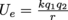goes to 0. This is a consistent definition with our formulas for force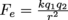approaches 0 as r → ∞) and electric field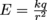also approaches 0 as r → ∞).

Consistency is good. Not "we've won the lottery, gotten an A on our physics test, and found a lucky penny" kinds of good, but we'll still take it.

### Common Mistakes

The formulas for Fe, E, V, and Ue all look very similar—an easy way to make sure you're using the right one is to check the units. Forces (Fe) are in N, electric fields (E) are in N/C, electric potentials (V) are in V or J/C, and electric potential energies are in J.

### Brain Snack

If you thought the platypus was impressive, well, it's not the only electric animal out there. Some fish, like the electric eel, are capable of creating huge potential differences on their bodies, which they can discharge to occasionally disastrous effect (500 V of hey-I'm-gonna-eat-you tends not to be too fun for that many other animals).